Home

# A GENERALISATION OF THE FROBENIUS RECIPROCITY THEOREM

## Abstract

Let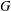$G$ be a locally compact group and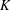$K$ a closed subgroup of$G$ . Let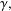$\unicode[STIX]{x1D6FE},$$\unicode[STIX]{x1D70B}$ be representations of$K$ and$G$ respectively. Moore’s version of the Frobenius reciprocity theorem was established under the strong conditions that the underlying homogeneous space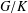$G/K$ possesses a right-invariant measure and the representation space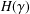$H(\unicode[STIX]{x1D6FE})$ of the representation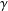$\unicode[STIX]{x1D6FE}$ of$K$ is a Hilbert space. Here, the theorem is proved in a more general setting assuming only the existence of a quasi-invariant measure on$G/K$ and that the representation spaces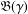$\mathfrak{B}(\unicode[STIX]{x1D6FE})$ and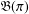$\mathfrak{B}(\unicode[STIX]{x1D70B})$ are Banach spaces with$\mathfrak{B}(\unicode[STIX]{x1D70B})$ being reflexive. This result was originally established by Kleppner but the version of the proof given here is simpler and more transparent.

## References

Hide All
MathJax
MathJax is a JavaScript display engine for mathematics. For more information see http://www.mathjax.org.

# A GENERALISATION OF THE FROBENIUS RECIPROCITY THEOREM

## Metrics

### Full text viewsFull text views reflects the number of PDF downloads, PDFs sent to Google Drive, Dropbox and Kindle and HTML full text views.

Total number of HTML views: 0
Total number of PDF views: 0 *Loading metrics...

### Abstract viewsAbstract views reflect the number of visits to the article landing page.

Total abstract views: 0 *Loading metrics...

* Views captured on Cambridge Core between <date>. This data will be updated every 24 hours.

Usage data cannot currently be displayed